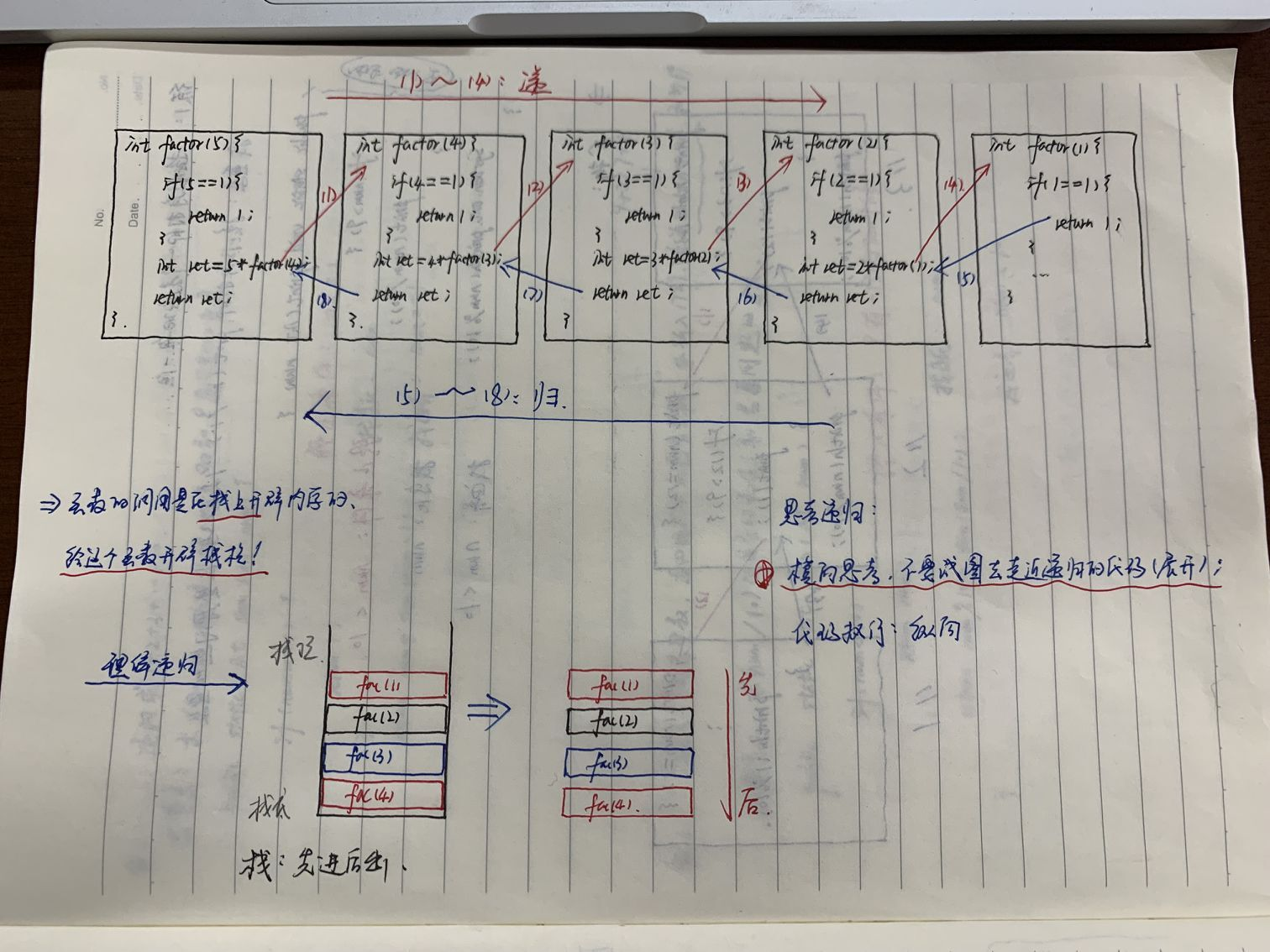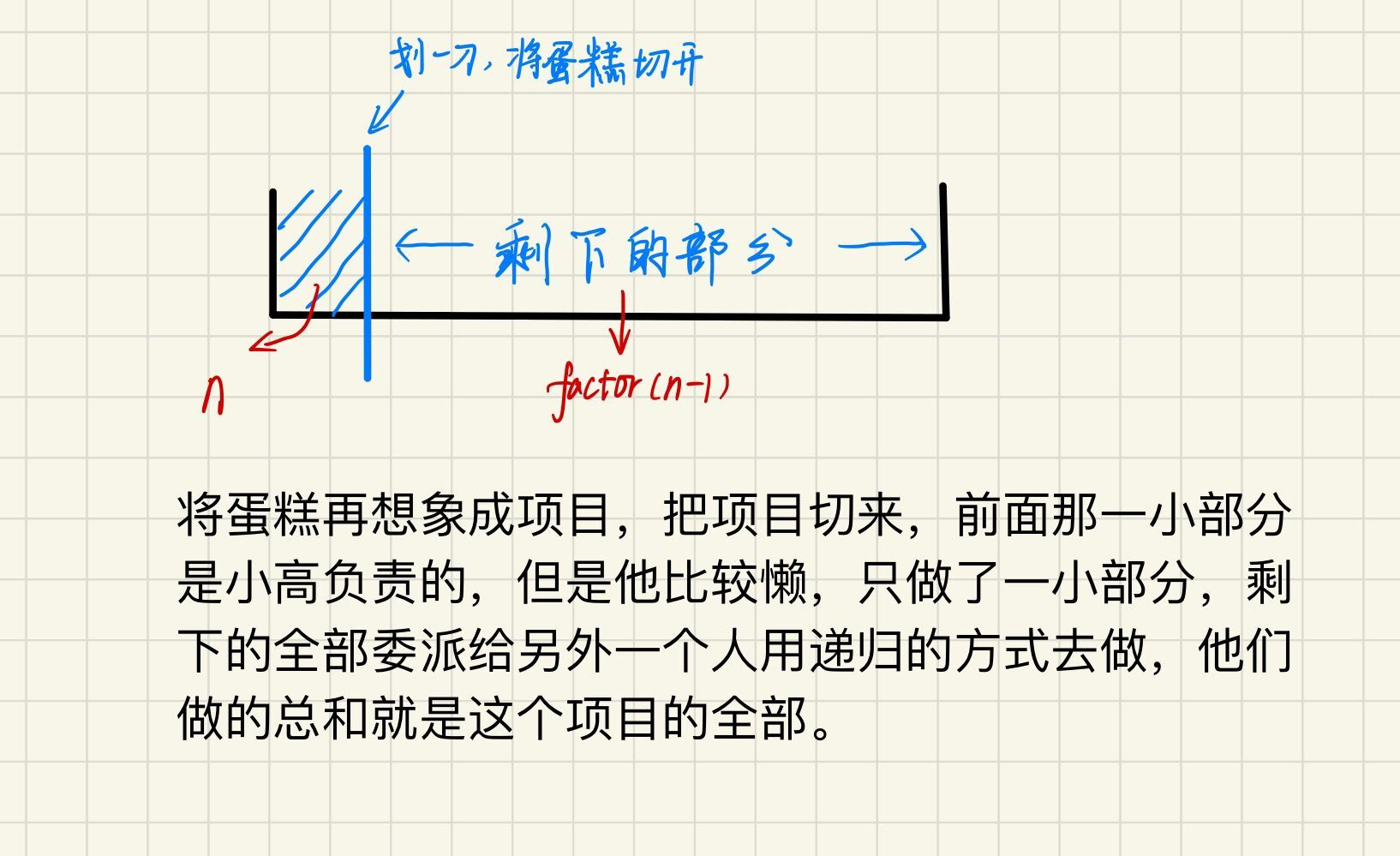# 蓝桥杯算法竞赛系列第二章——深入理解重难点之递归（上）

1、递归定义

2、递归需要满足的三个条件

3、递归函数

1、大招：递归“三段论式”设计经验

2、练习策略

1、求n的阶乘

2、递归求1+2+...+10

3、返回各位数字之和

4、按顺序打印整数i~j

5、对数组arr所有元素求和

【声明】：为了让更多的铁汁理解递归，笔者在前面的引入部分赘述可能过长，请铁汁们多点耐心哦，后面有大招。

【前言】：很多人都认为“递归”是语言学习中最难理解的内容之一，当然笔者也是这么认为的，哈哈，但是既然笔者已经将文章发布出来了，自然是有了充分的准备，所以，铁汁们不用紧张，看到最后你会发现，递归其实是一个很自然的东西。

# 一、递归是什么？

f(n) = f(n-1) + 1 其中，f(1)=1
f(n) 表示你想知道自己在哪一排，f(n-1) 表示前面一排所在的排数，f(1)=1 表示第一排的人知道自己在第一排。

# 二、如何理解“递归”？

## 1、递归定义

A经理：“这事不归我管，去找B经理。” 于是你去找B经理。

B经理：“这事不归我管，去找A经理。”于是你又回到了A经理这儿。

（1）1是正整数。

（2）如果n是正整数，那么n + 1也是正整数。

（3）只有通过（1）、（2）定义出来的才是正整数。（这里牺牲一点严密性，换来的是更通俗易懂的表达方式）

## 2、递归需要满足的三个条件

1. 一个问题的解可以分解为几个子问题的解

2. 这个问题与分解之后的子问题，除了数据规模不同，求解思路完全一样

3. 存在递归终止条件

## 3、递归函数

``````#include<stdio.h>

int factor(int n)
{
if (n == 1)
{
return 1;
}
return n * factor(n - 1);
}

int main()
{
int n = 5;

int ret = factor(n);

printf("%d\n", ret);

return 0;
}``````

# 三、怎么玩转递归

## 1、大招：递归“三段论式”设计经验

对于递归这种折磨人的知识，有人两三天就会了，有人三五年也不会。主要看你悟不悟得透，能不能找到对于递归的感觉。 三段论中的找重复，这个步骤是最重要而且还是最需要感觉的，不过你要相信Practice makes prefect！踏踏实实，多做，多练，多敲。 在找边界设计出口时，最好写在函数的开头。

# 四、精选练习题讲解

## 1、求n的阶乘

### 三段论：

找重复：n * (n - 1)的阶乘，求(n - 1)的阶乘是原问题的重复，规模更小，是原问题的子问题。 找变化：找重复中的变化的量作为参数。本题中很明显是n在变化，所以函数定义过程中的形参是n. 找边界：也就是出口的判断，什么时候让函数停止。显然本题中当n == 1时结束。

### 代码执行

``````#include<stdio.h>

//本题中的变化量是n，所以用作形式参数
int factor(int n)
{
//出口的判断，最好写在函数的开头，本题中当n == 1时就意味着到边界了，应该终止程序
if (n == 1)
{
return 1;
}
//(n - 1)的阶乘是原问题的重复，是其子问题，规模更小
return n * factor(n - 1);
}

int main()
{
int n = 5;

int ret = factor(5);

printf("%d\n", ret);

return 0;
}``````## 2、递归求1+2+...+10

### 代码执行

``````//递归求1+2+3+...+10
#include<stdio.h>
//很显然num是不断变化的，所以用作函数的形参
int sum(int num)
{
//找边界
if (num == 1)
{
return 1;
}
//1~(num - 1)是原问题的重复，规模更小，是原问题的子问题
return num + sum(num - 1);
}

int main()
{
int n = 10;

int ret = sum(n);

printf("%d\n", ret);

return 0;
}
``````

## 3、返回各位数字之和

### 代码执行

``````#include<stdio.h>
int sum(int num)
{
if (num < 10)
{
return num;
}
return num % 10 + sum(num / 10);
}

int main()
{
int num = 1729;

printf("%d\n", sum(num));

return 0;
}``````

## 4、按顺序打印整数i~j

### 代码执行

``````#include<stdio.h>

void f2(int i, int j)
{
if (i > j)
{
return;
}
printf("%d ", i);
f2(i + 1, j);
}

int main()
{
f2(2, 9);

return 0;
}``````

## 5、对数组arr所有元素求和

### 代码执行

``````public class Recursion {

public static int f3(int[] arr, int begin) {
if(begin == arr.length - 1) {
return arr[begin];
}
return arr[begin] + f3(arr, begin + 1);
}
public static void main(String[] args) {

int[] arr = {1,3,4,6,7,8,9};
int ret = f3(arr, 0);
System.out.println(ret);
}
}``````

# 五、思考题

https://blog.csdn.net/weixin_57544072/article/details/120798996utm_source=app&app_version=4.16.0

# 六、蓝桥结语：遇见蓝桥遇见你，不负代码不负卿